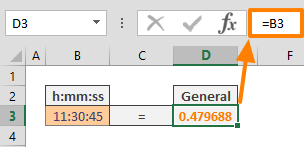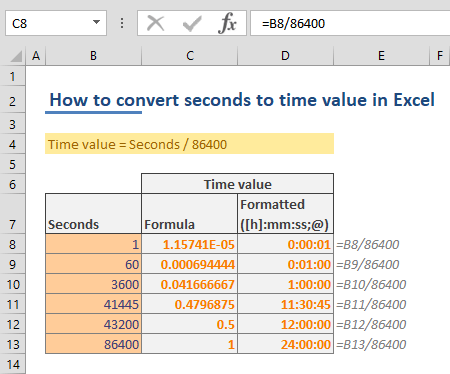When it comes to date and time values, Excel evaluates them a bit differently than how we process them. For Excel, date and time values are numbers in a date or time display. Although this behavior allows making date and time calculations easier, it can be helpful to know your way around converting time values like seconds, into Excel time format. In this guide, we are going to show you how to convert seconds to time value in Excel.

## Basics of date and time values in Excel

Excel stores date and time as numbers. Excel assumes that the date 1/1/1900 is “1″, and increases this number by 1 for each day. In a similar way, the time values can be any number between 0 and 0.999999999. For example; 6:00 AM is equal to 0.25.

You can see the numeric value of a date or time value by changing number format of a cell contains a date or number.## Converting seconds into time values in Excel

Since time values are numbers in Excel, there are basically no restrictions in using a mathematical operation to convert seconds to time values in Excel. As mentioned in the previous section, Excel assumes that time values are distributed in 1 day. So, we know that 1 day is equal to to 24 hours, 1 hour is equal to 60 minutes and 1 minute is equal to 60 seconds. With this idea, 1 day is equal to 86400 seconds. All you need to do is to divide the seconds value by 86400 to convert seconds into 1-day base.

Time value = Seconds / 86400

Here is table that shows what you would see when you divide some numbers by 86,400. Also, the third column shows the same value in a time number format "[h]:mm:ss;@".